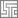# Page 1: Vector

Home » Knowledge Base » LS-Prepost » Pages » Page1 » Page 1: Vector

•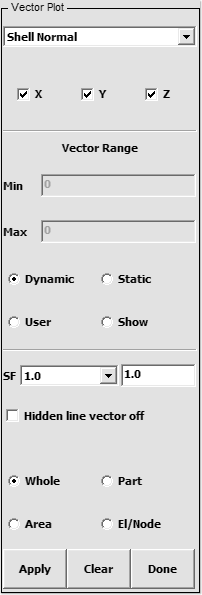• Figure 1 – Vector Interface

## Purpose:

This interface is used to display normal vectors for any element in the model.

## Controls:

• Select Vector Type: Choose from list
• (Shell Normal / Displacement / Velocity / Prin. Stress / Prin Strain / P. Inplane Strain / Heat Flux)

•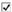X – Toggle X component of vector
•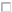Y – Toggle Y component of vector
•Z – Toggle Z component of vector

• Vector Range: Enter minimum and maximum values for vector range
• (Press enter to accept values)

•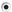Dynamic – A set of min/max ranges is computed for each time state
•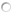Static – A constant min/max range is computed using all time states
•User – Range set by user, enter min/max values above
•Show – Shows elements within the range entered above

• SF: Enter scale factor for vector plot
•Hidden line vector off – Switch off hidden line for vectors
• (If a vector is behind part of the model it will not be shown, check this option to display the vector)

•Whole – Apply vector plot to whole model
•Part – Pick parts for vector plot
•Area – Define an area for vector plot
•El/Node – Pick an element or node for vector plot

Apply vector plot

Clear vector plot

Exit Vector Plot interface